Courses

# Simple Stresses (Part - 3) Mechanical Engineering Notes | EduRev

## Mechanical Engineering : Simple Stresses (Part - 3) Mechanical Engineering Notes | EduRev

The document Simple Stresses (Part - 3) Mechanical Engineering Notes | EduRev is a part of Mechanical Engineering category.
All you need of Mechanical Engineering at this link: Mechanical Engineering

Stress at a point—its implication in design

The state of stress at a point is given by nine stress components as shown in figure 2.1.6.1 and this is represented by the general matrix as shown below.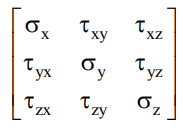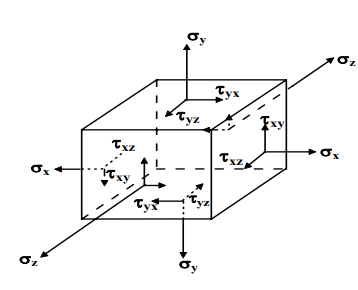2.1.6.1F- Three dimensional stress field on an infinitesimal element.

Consider now a two dimensional stress element subjected only to shear stresses. For equilibrium of a 2-D element we take moment of all the forces about point A ( figure-2.1.6.2) and equate to zero as follows: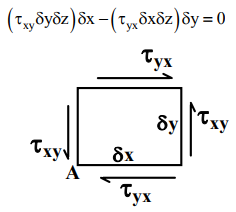2.1.6.2F- Complimentary shear stresses on a 2-D element.

This gives τxyyx indicating that τxy and τyx are complimentary. On similar arguments we may write τyzzy and τzxxz . This means that the state of stress at a point can be given by six stress components only. It is important to understand the implication of this state of stress at a point in the design of machine elements where all or some of the stresses discussed above may act.

For an example, let us consider a cantilever beam of circular cross-section subjected to a vertical loading P at the free end and an axial loading F in addition to a torque T as shown in figure 2.1.6.3. Let the diameter of cross-section and the length of the beam be d and L respectively.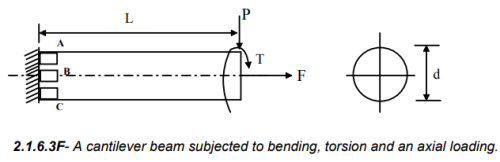The maximum stresses developed in the beam are :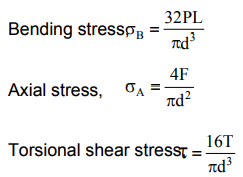It is now necessary to consider the most vulnerable section and element. Since the axial and torsional shear stresses are constant through out the length, the most vulnerable section is the built-up end. We now consider the three elements A, B and C. There is no bending stress on the element B and the bending and axial stresses on the element C act in the opposite direction. Therefore, for the safe design of the beam we consider the stresses on the element A which is shown in figure 2.1.6.4.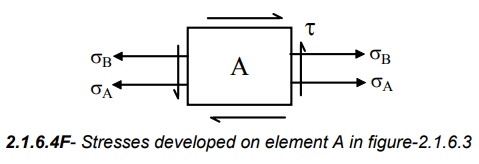Principal stresses and maximum shear stresses can now be obtained and using a suitable failure theory a suitable diameter of the bar may be obtained.

Offer running on EduRev: Apply code STAYHOME200 to get INR 200 off on our premium plan EduRev Infinity!

,

,

,

,

,

,

,

,

,

,

,

,

,

,

,

,

,

,

,

,

,

;